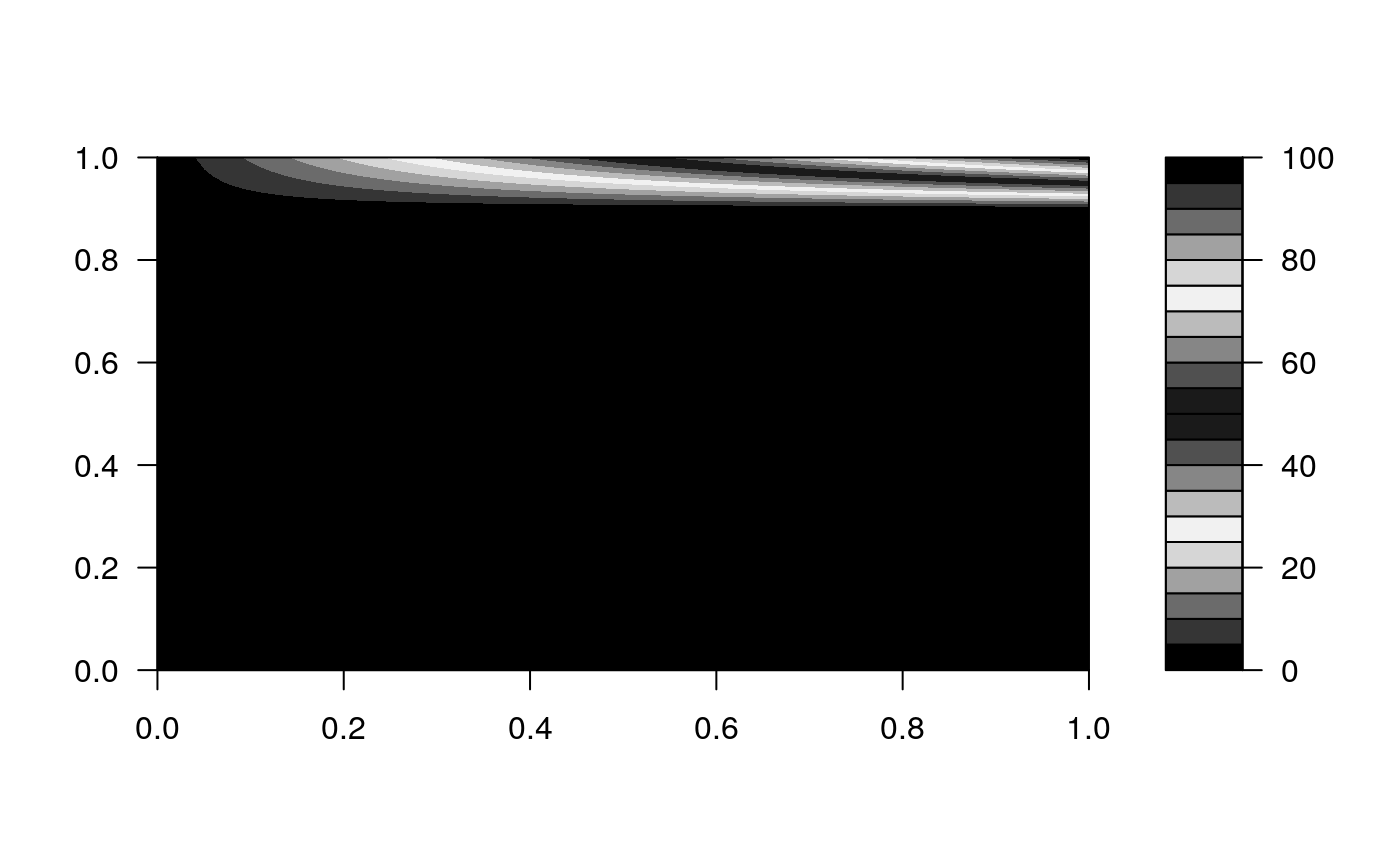emis_det returns deterioration factors. The emission factors comes from the guidelines for developing emission factors of the EMEP/EEA air pollutant emission inventory guidebook http://www.eea.europa.eu/themes/air/emep-eea-air-pollutant-emission-inventory-guidebook This function subset an internal database of emission factors with each argument

emis_det(po, cc, eu, speed = Speed(18.9), km, verbose = FALSE,
show.equation = FALSE)

## Arguments

po Character; Pollutant "CO", "NOx" or "HC" Character; Size of engine in cc converin "<=1400", "1400_2000" or ">2000" Character; Euro standard: "I", "II", "III", "III", "IV", "V", "VI", "VIc" Numeric; Speed to return Number of emission factor and not a function. It needs units in km/h Numeric; accumulated mileage in km. Logical; To show more information Option to see or not the equation parameters

## Value

It returns a numeric vector representing the increase in emissions due to normal deterioring

## Note

The deterioration factors functions are available for technologies euro "II", "III" and "IV". In order to cover all euro technologies, this function assumes that the deterioration function of "III" and "IV" applies for "V", "VI" and "VIc". However, as these technologies are relative new, accumulated milage is low and hence, deteerioration factors small.

## Examples

{
data(fkm)
pckm <- fkm[](1:24); pckma <- cumsum(pckm)
km <- units::set_units(pckma[1:11], km)
# length eu = length km = 1
emis_det(po = "CO", cc = "<=1400", eu = "III", km = km, show.equation = TRUE)
# length eu = length km = 1, length speed > 1
emis_det(po = "CO", cc = "<=1400", eu = "III", km = km, speed = Speed(1:10))
# length km != length eu error
# (cod1 <- emis_det(po = "CO", cc = "<=1400", eu = c("III", "IV"), speed = Speed(30),
# km = km))
# length eu = 1 length km > 1
emis_det(po = "CO", cc = "<=1400", eu = "III", km = km)
# length eu = 2, length km = 2 (if different length, error!)
(cod1 <- emis_det(po = "CO", cc = "<=1400", eu = c("III", "IV"), km = km[4:5]))
# length eu = 2, length km = 2, length speed > 1
(cod1 <- emis_det(po = "CO", cc = "<=1400", eu = c("III", "IV"), speed = Speed(0:130),
km = km[4:5]))
euros <- c("V","V","V", "IV", "IV", "IV", "III", "III", "III", "III")
# length eu = 2, length km = 2, length speed > 1
(cod1 <- emis_det(po = "CO", cc = "<=1400", eu = euros, speed = Speed(1:100),
km = km[1:10]))
cod1 <- as.matrix(cod1[, 1:11])
filled.contour(cod1, col = cptcity::cpt(6277, n = 20))
filled.contour(cod1, col = cptcity::lucky(n = 19))
euro <- c(rep("V", 5), rep("IV", 5), "III")
euros <- rbind(euro, euro)
(cod1 <- emis_det(po = "CO", cc = "<=1400", eu = euros, km = km))
}#> b = 7.13e-06, c = 0.769, MAX MC URBAN = 1.91,
#> f = 1.5e-06, g = 0.955, MAX MC ROAD = 1.2
#> V < 19: MC_URBAN = b*km + c
#> V >= 63: MC_ROAD = f*km + g
#> 19 <= V < 63: MC_URBAN + (V - 19)*(MC_ROAD - MC_URBAN)/44#> Colour gradient: ocal_pastel_blue, number: 6293#>   V1 V2       V3       V4       V5      V6       V7       V8       V9      V10
#> 1  1  1 1.038558 1.135773 1.235658 1.33765 1.441215 1.545847 1.651069 1.756434
#> 2  1  1 1.038558 1.135773 1.235658 1.33765 1.441215 1.545847 1.651069 1.756434
#>       V11       speed
#> 1 1.86152 18.9 [km/h]
#> 2 1.86152 18.9 [km/h]